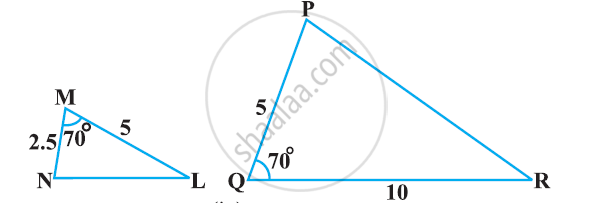# State which pair of triangles in the following figure are similar? Write the similarity criterion used by you for answering the question and also write the pairs of similar triangles in the symbolic form - Mathematics

State which pair of triangles in the following figure are similar? Write the similarity criterion used by you for answering the question and also write the pairs of similar triangles in the symbolic form:#### Solution

In ∆ MNL and ∆ QPR, we observe that,

(MN)/(QP) = (ML)/(QR) = 1/2

M = Q = 70

MNL ~ QPR  [By SAS similarity criterion]

Concept: Criteria for Similarity of Triangles
Is there an error in this question or solution?

#### APPEARS IN

NCERT Class 10 Maths
Chapter 6 Triangles
Exercise 6.3 | Q 1.4 | Page 139